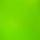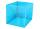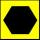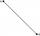# Triangle - math word problems

1. Area of RT 2Calculate the area of right triangle whose legs have a length 5.8 cm and 5.8 cm.
2. ArcCalculate span of the arc, which is part of a circle with diameter d = 20 m and its height is 6 m.
3. RailwaysRailways climb 7.4 ‰. Calculate the height difference between two points on the railway distant 3539 meters.
4. RT and circlesSolve right triangle if the radius of inscribed circle is r=9 and radius of circumscribed circle is R=23.
5. Square2Side of the square is a = 6.2 cm, how long is its diagonal?I have homework. The cube has an edge 7 cm long and I must find wall and body diagonal.
7. Height UTHow long is height in the equilateral triangle with a side b = 43?
8. TriangleCalculate the area of ​​the triangle ABC if b = c = 17 cm, R = 19 cm (R is the circumradius).
9. HexagonDraw a regular hexagon inscribed in a circle with radius r=8 cm. What is its perimeter?
10. SquareRectangular square has side lengths 183 and 244 meters. How many meters will measure the path that leads straight diagonally from one corner to the other?Average climb of the road is given by ratio 1:15. By what angle road average climb?
12. TriangleTriangle KLM is given by plane coordinates of vertices: K[11, -10] L[10, 12] M[1, 3]. Calculate its area and its interior angles.
13. Greatest angleCalculate the greatest triangle angle with sides 197, 208, 299.
14. SimilarityAre two right triangles similar to each other if the first one has a acute angle 70° and second one has acute angle 20°?
15. SAS triangleThe triangle has two sides long 7 and 19 and included angle 36°. Calculate area of this triangle.
16. PrismRight angle prism, whose base is right triangle with leg a = 3 cm and hypotenuse c = 13 cm has same volume as a cube with an edge length of 3 dm. a) Determine the height of the prism b) Calculate the surface of the prism c) What percentage of the cub
17. Reverse Pythagorean theoremGiven are lengths of the sides of the triangles. Decide which one is rectangular: Δ ABC: 77 dm, 85 dm, 36 dm ? Δ DEF: 55 dm, 82 dm, 61 dm ? Δ GHI: 24 mm, 25 mm, 7 mm ? Δ JKL: 32 dm, 51 dm, 82 dm ? Δ MNO: 51 dm, 45 dm, 24 dm
18. VectorCalculate length of the vector v⃗ = (9.75, 6.75, -6.5, -3.75, 2).
19. SegmentCalculate the length of the segment AB, if the coordinates of the end vertices are A[10, -4] and B[5, 5].
20. AnglesThe outer angle of the triangle ABC at the vertex A is 114°12'. The outer angle at the vertex B is 139°18'. What size is the internal angle at the vertex C?

Do you have an interesting mathematical word problem that you can't solve it? Submit math problem, and we can try to solve it.

We will send a solution to your e-mail address. Solved examples are also published here. Please enter the e-mail correctly and check whether you don't have a full mailbox.

Please do not submit problems from current active competitions such as Mathematical Olympiad, correspondence seminars etc...